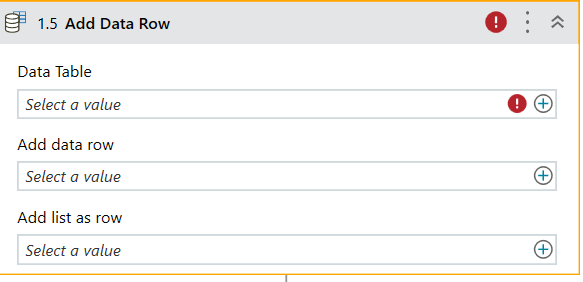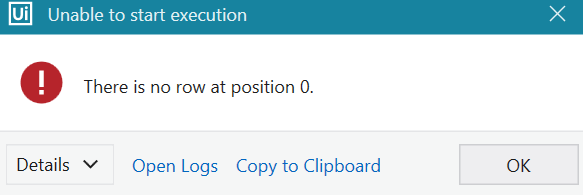# Text file error to convert into excel

Hi,
I want to convert text file into excel. I have succeed to convert it but i have few problem.

1. For the first 3 column name is combine into 1 column which i want it to be 3 different column. Then, after the first column suddenly there is few more new column insert

I think that this is the only way is i add one template file that has old data. So i need to replace the new data with the new text file thus my text file that converted into excel can follow the template that i want

May i know how to make the text file follow my previous template so that when the text file convert into excel it will follow as i want it to be?

@Nur_Amin
There could be issue with delimiter in that text file, which is why columns are getting combine.
Is it possible for you to give us a sample Input and output data ?

i have already discover the solution but encounter another problem. can help me on this concatenate. i share with u the sample excel that i want

i want to concatenate two rows in every column, i already done write data table to excel
CONCATENATE EXAMPLE.xlsx (8.7 KB)

so your requirement is limited to 2 rows only or there could more than 2 rows as well…?
If more than 2 rows, what should be done…? Ignore rest of rows ?

yes only two row.

In add datarow>ArrayRow> use below code

``````{dt_Input(0)(0).ToString + dt_Input(1)(0).ToString,dt_Input(0)(1).ToString+dt_Input(1)(1).ToString,dt_Input(0)(2).ToString + dt_Input(0)(2).ToString}
``````

Method2:

In add datarow>ArrayRow> use below code

``````{String.Join("",(from rw in dt_Input select x =rw(0).ToString)),String.Join("",(from rw in dt_Input select x =rw(1).ToString)),String.Join("",(from rw in dt_Input select x =rw(3).ToString))}
``````

These examples are for 3 columns only. If required you can increase the columns.

for the code, what activity need i use?

i have choose add data row but where i need to doI did not knew that you are using StudioX
for StudioX use below line of code

`{dt_Input.Rows(0)(0).ToString + dt_Input.Rows(1)(0).ToString,dt_Input.Rows(0)(1).ToString+dt_Input.Rows(1)(1).ToString,dt_Input.Rows(0)(2).ToString + dt_Input.Rows(1)(2).ToString,dt_Input.Rows(0)(3).ToString + dt_Input.Rows(1)(3).ToString,dt_Input.Rows(0)(4).ToString + dt_Input.Rows(1)(4).ToString}`

Also check the attached file for your reference
Main.xaml (7.6 KB)

i cannot open. u have any idea on how i can fix this?

try this
Test.zip (49.9 KB)why appear like this when i try to run?

i got error. can u explain on the coding. im a bit hard to understand

Hello @Nur_Amin
We are doing the below steps

1. Reading the excel into dataTable dt_Input
2. Adding datarow in to dt_Input. For concatenate, we are directly referring the rows with the help of indexes.
3. Writing the dt_Input back to excel file.

Hope this clear out few things